# NCERT Exemplar Class 11 Physics Solutions for Chapter 5 - Laws Of Motion

NCERT exemplar Class 11 Physics Chapter 5 Laws of Motion is a supreme study material necessary for the students of CBSE Class11. In order to clear all their doubts in Chapter 5 Laws of Motion, it is very essential for the students to get familiar with all the questions and solutions provided here. This NCERT exemplar class 11 physics Chapter 5 pdf provides advance questions provided in NCERT exemplar books along with extra questions of laws of motion prepared by experts at BYJU’S and laws of motion entrance questions and answers. The Laws of Motion are some of the most basic laws that govern physics in our universe, which are based on the foundation of classical mechanics. Primarily there are three different laws that portray the relationship between forces and the body upon which these forces are acting. The three laws are:

1. First Law of Motion
2. Second Law of Motion
3. Third Law of Motion

Topics of Class 11 Physics Chapter 5 laws of motion

 Section Number Topic 1 Introduction 2 Aristotle’s fallacy 3 The law of inertia 4 Newton’s first law of motion 5 Newton’s second law of motion 6 Newton’s third law of motion 7 Conservation of momentum 8 Equilibrium of a particle 9 Common forces in mechanics 10 Circular motion 11 Solving problems in mechanics.

## Download PDF of NCERT Exemplar Class 11 Physics Chapter 5 Laws of Motion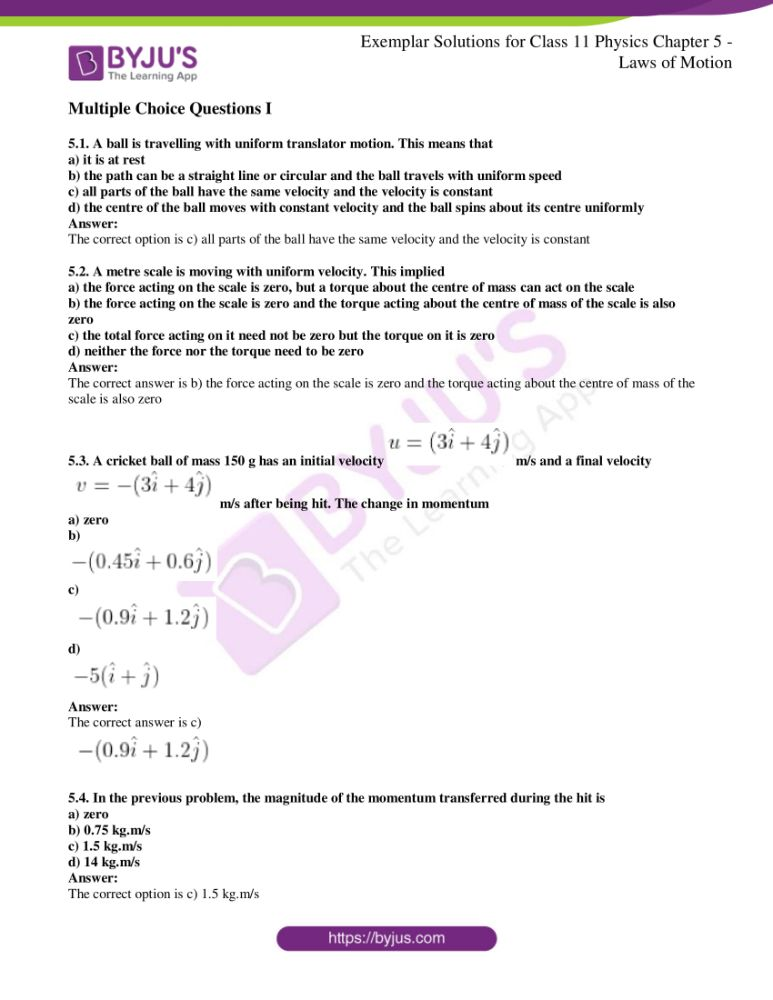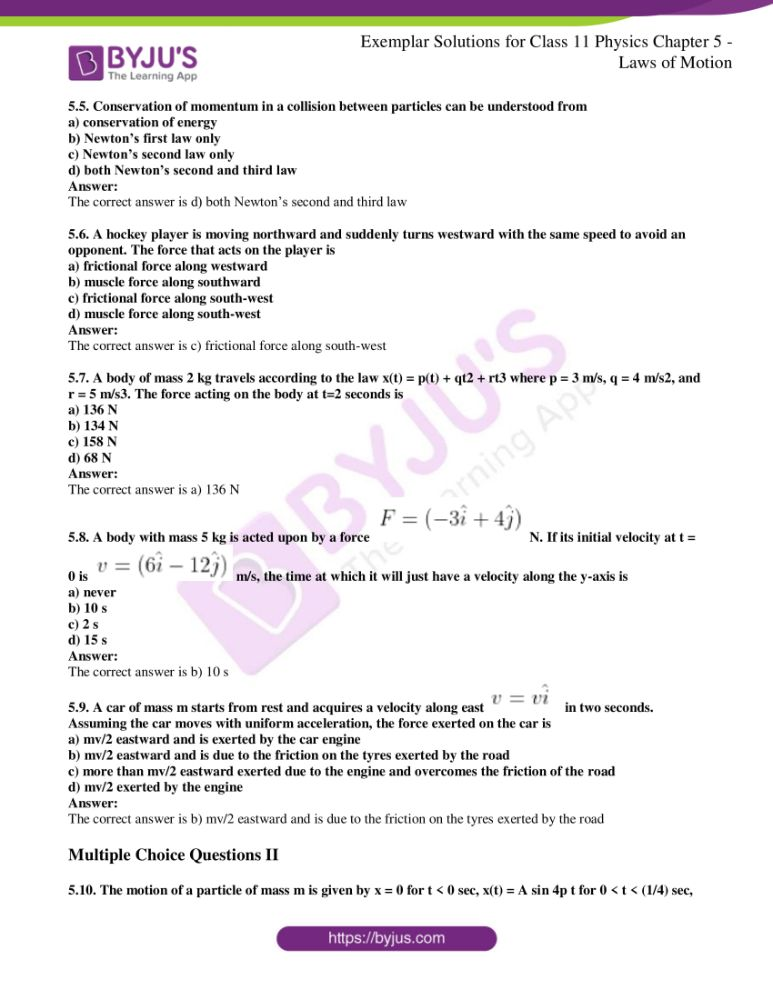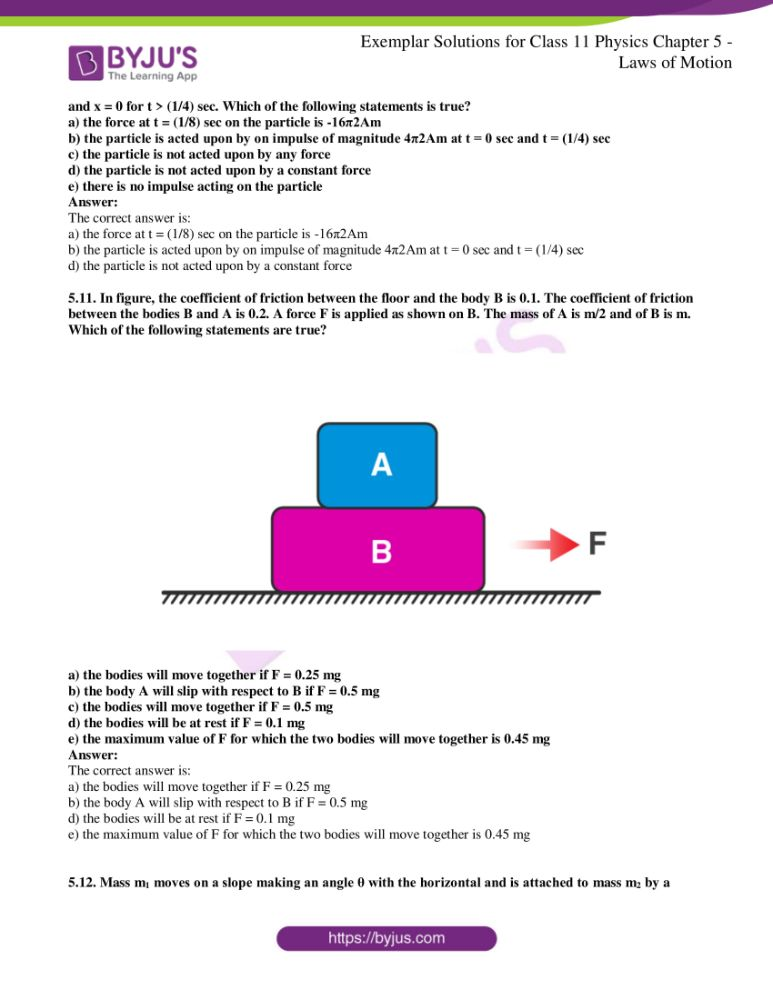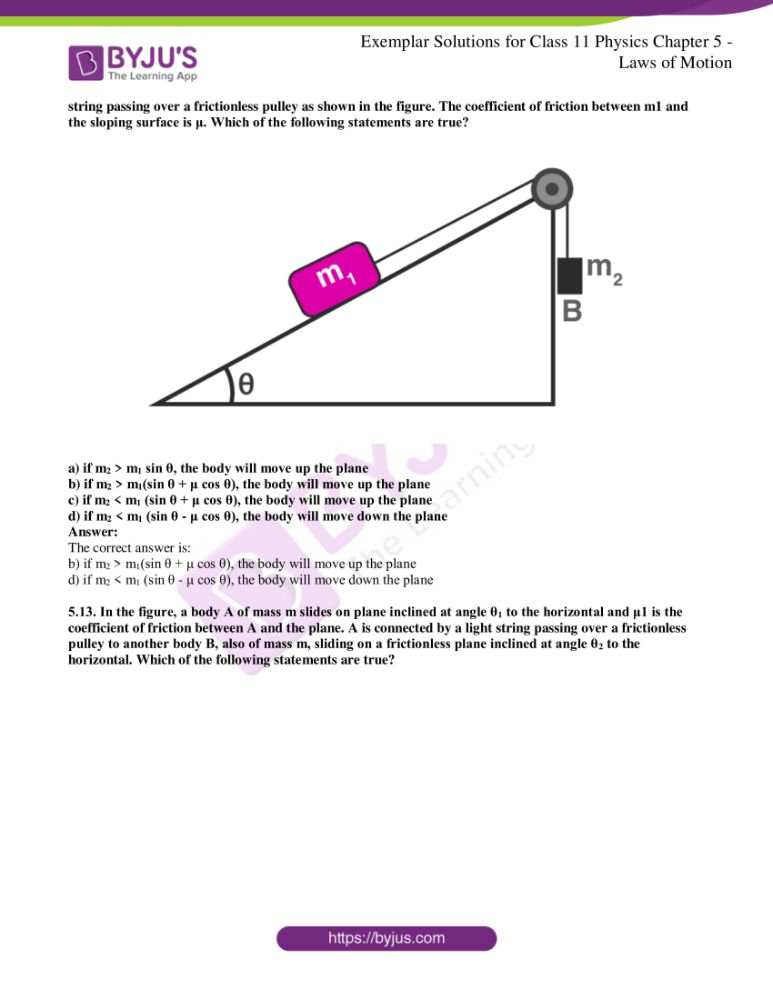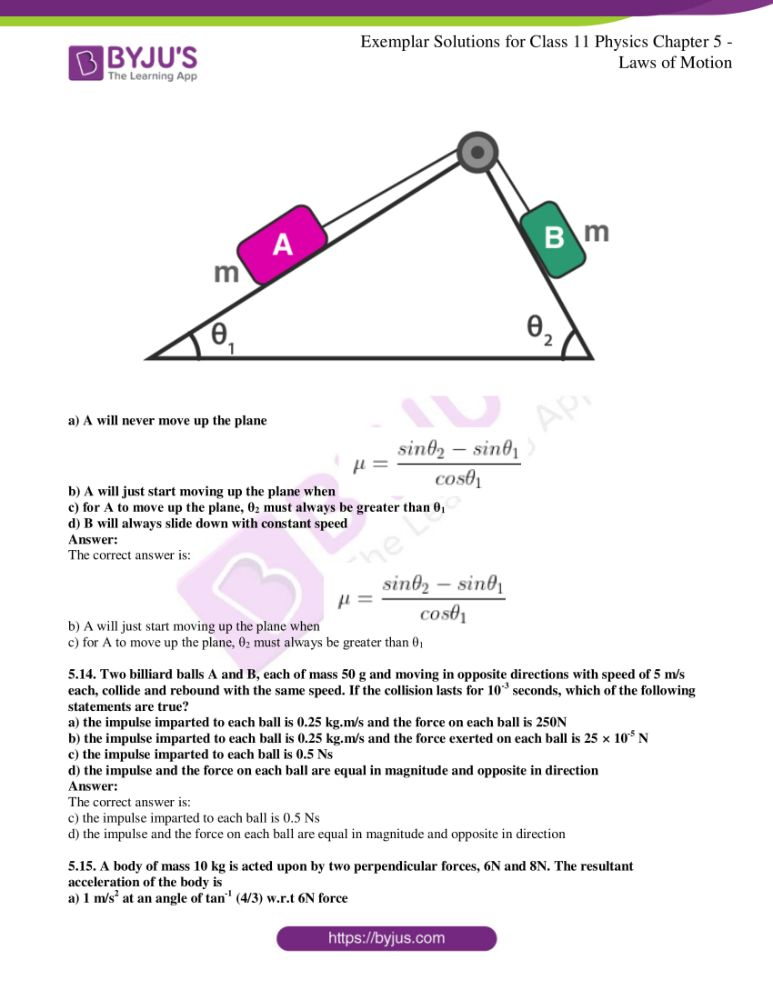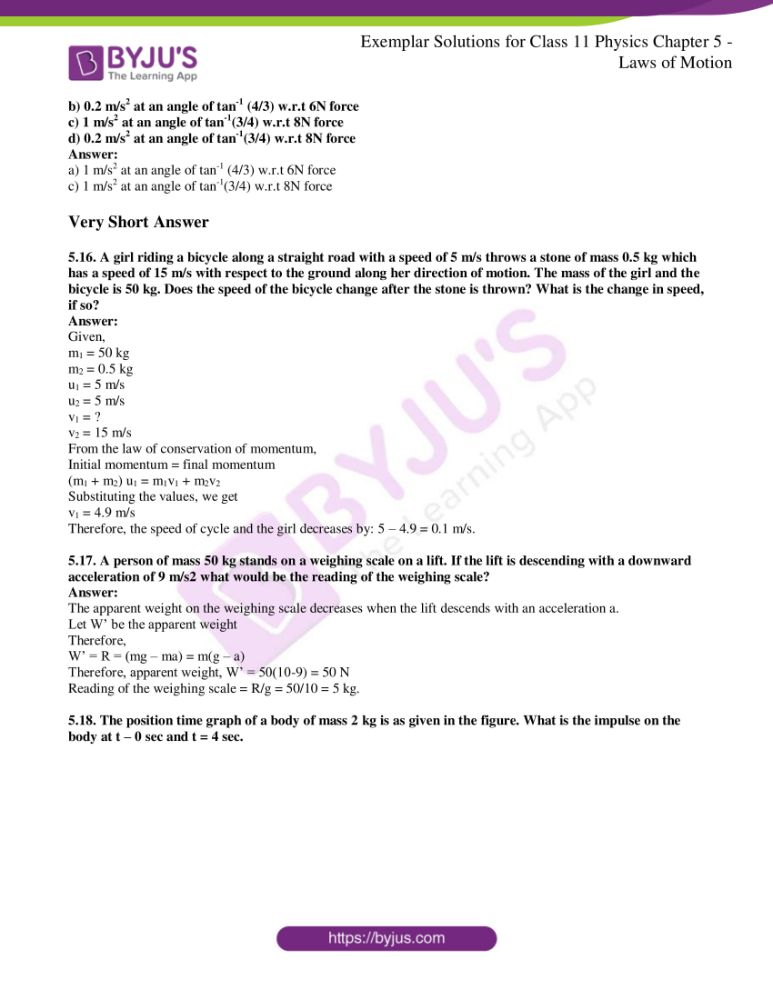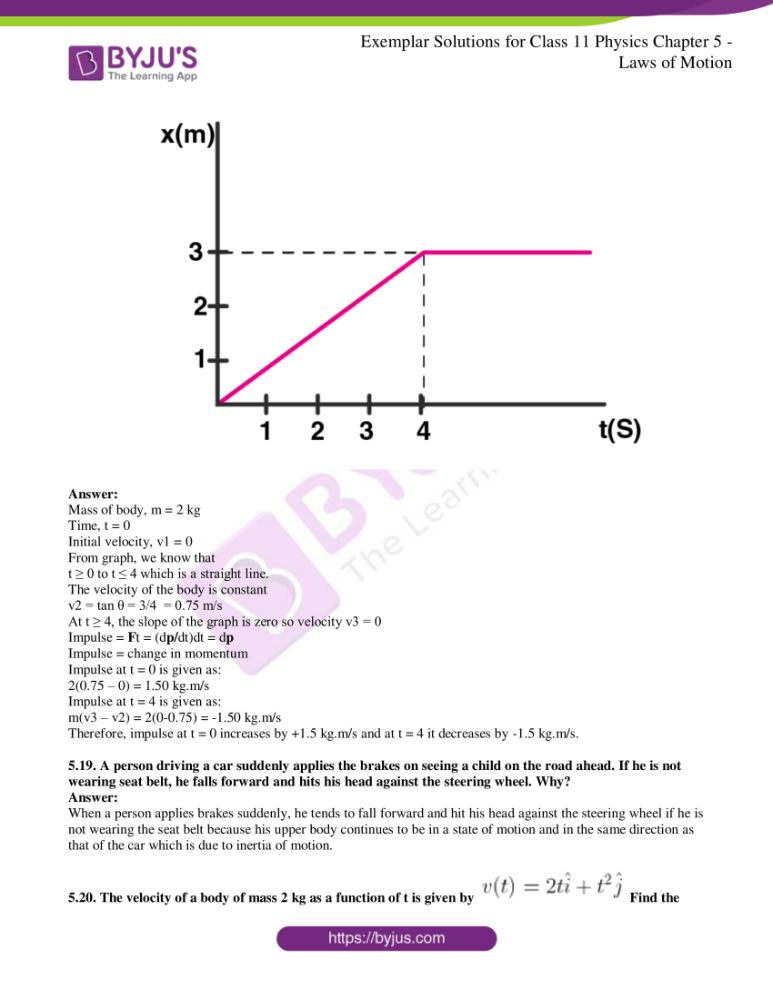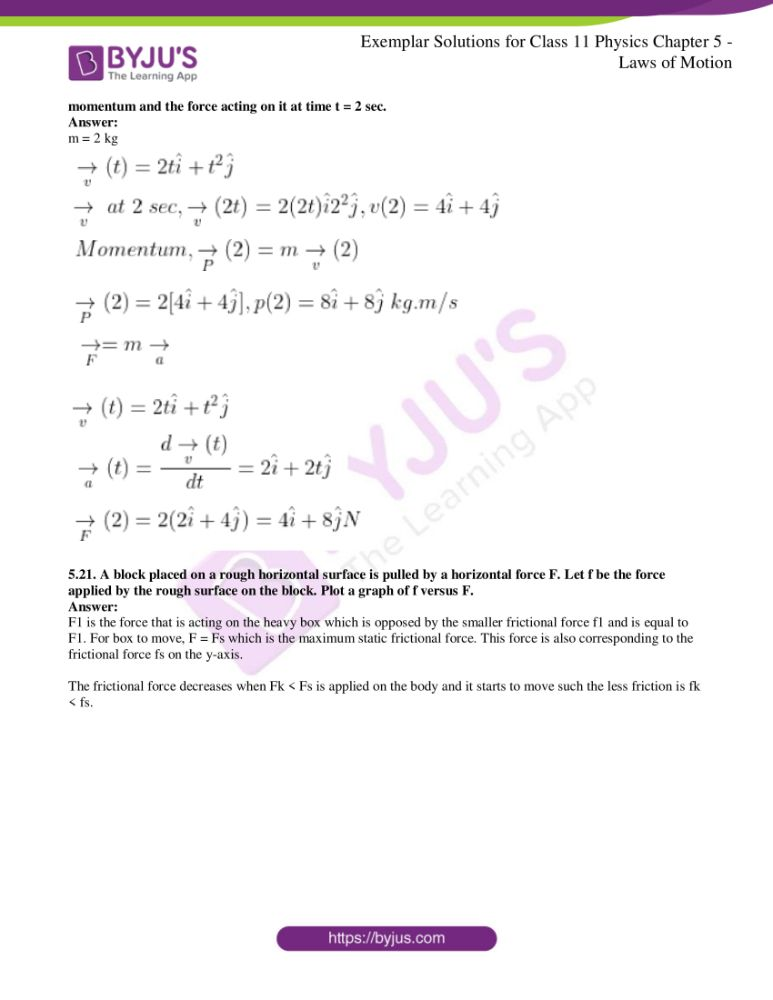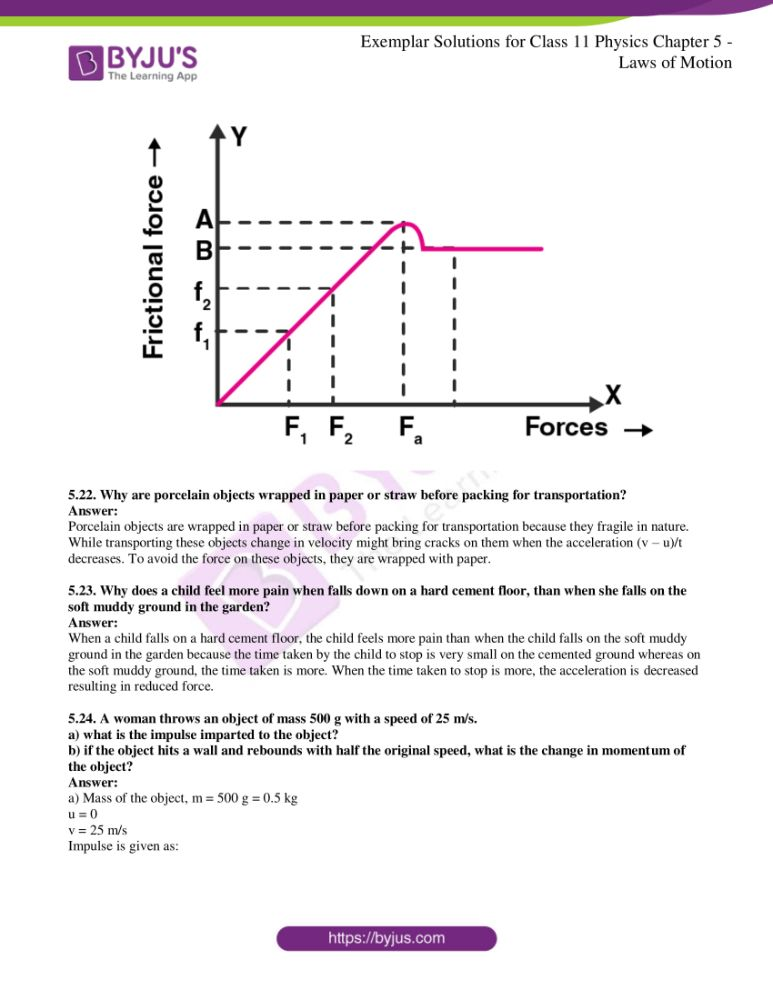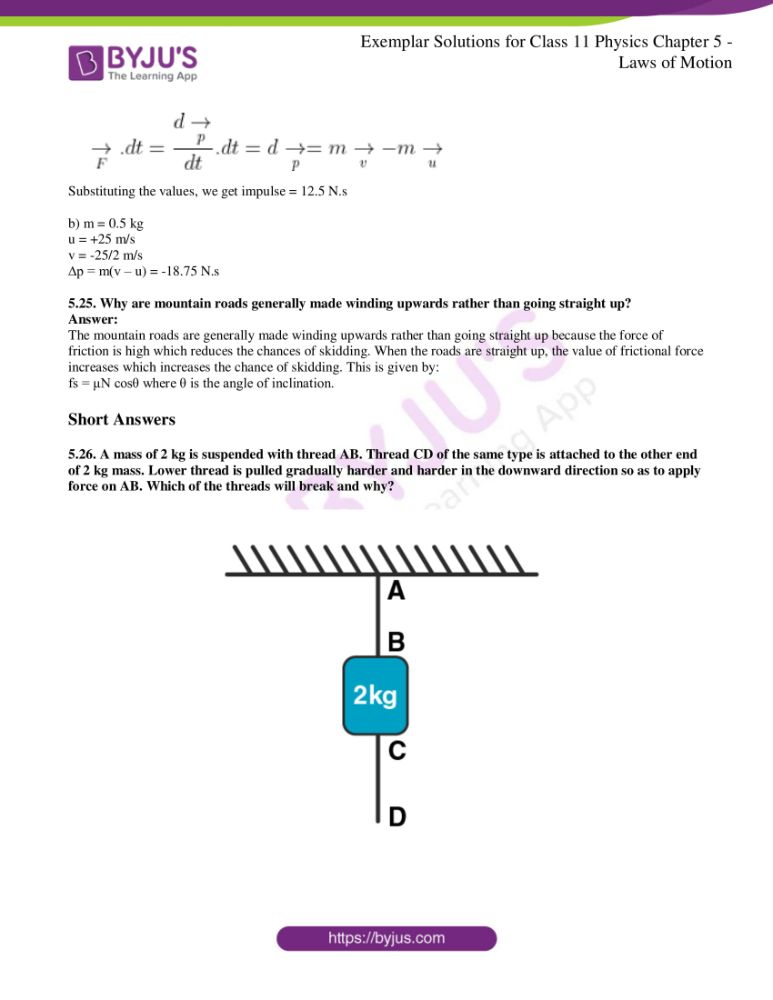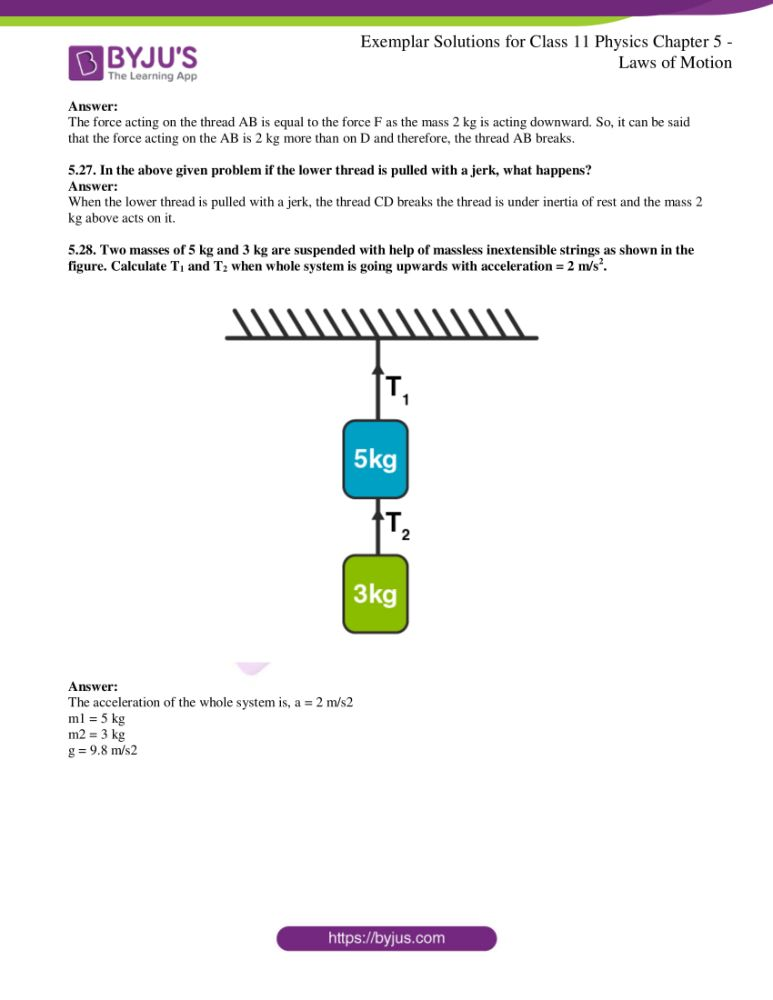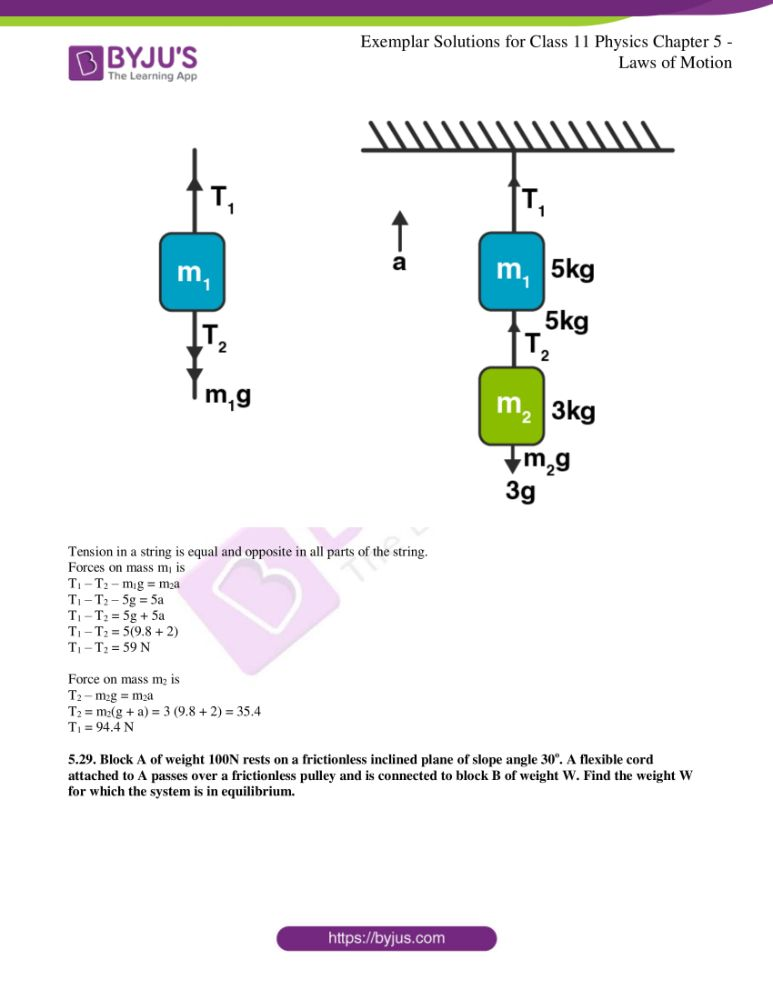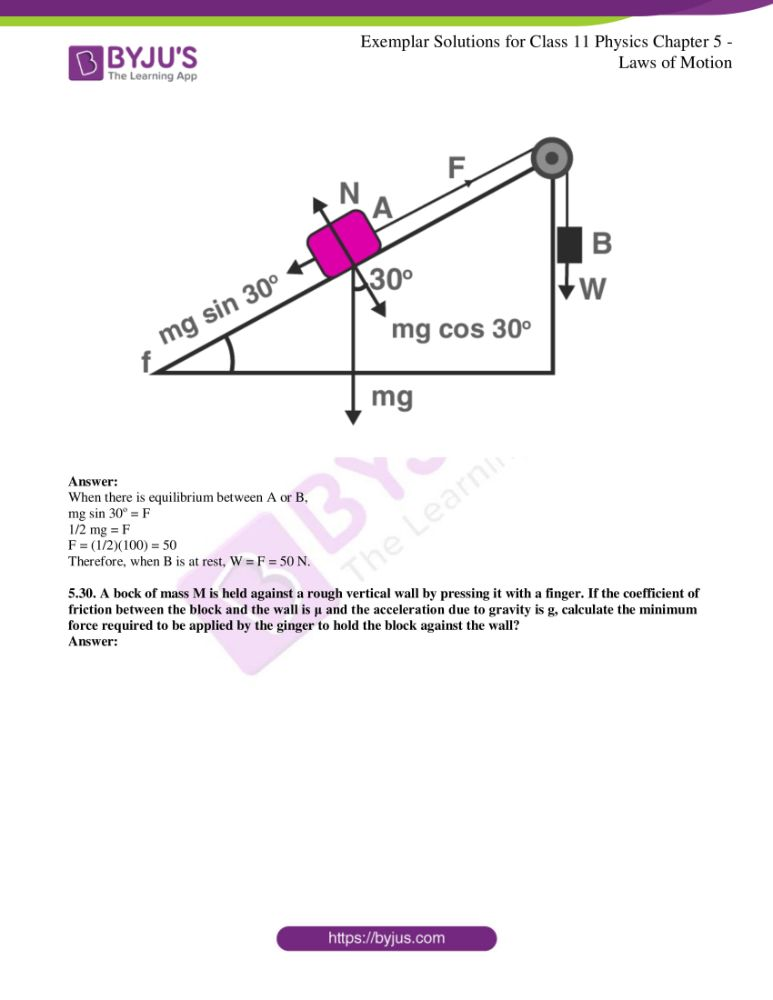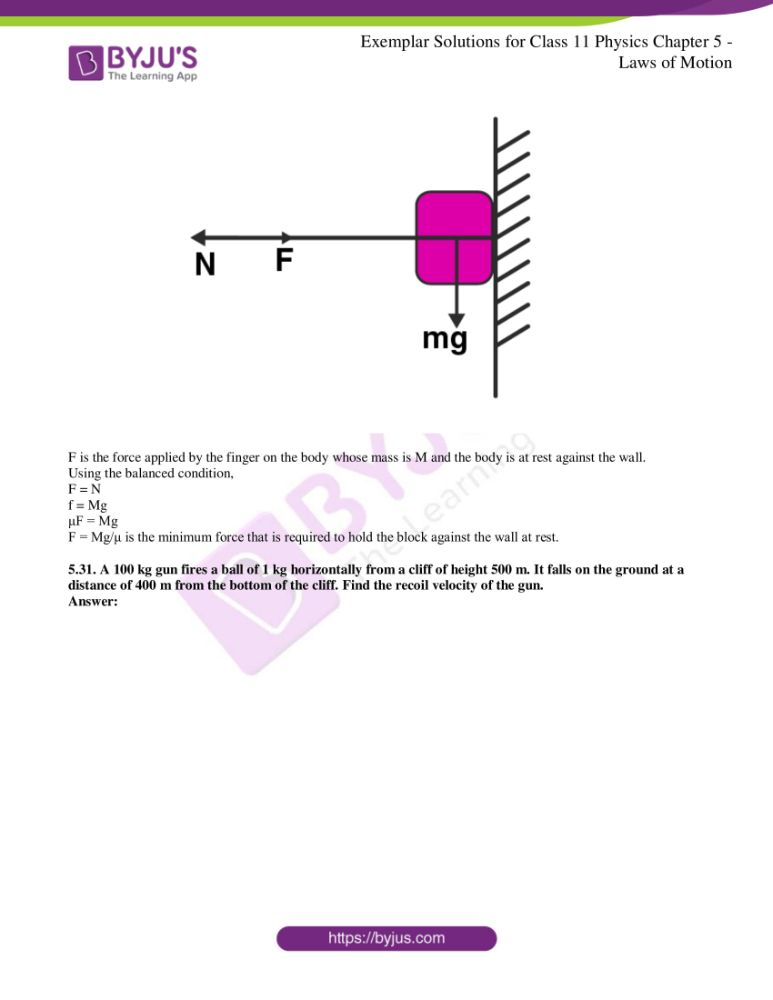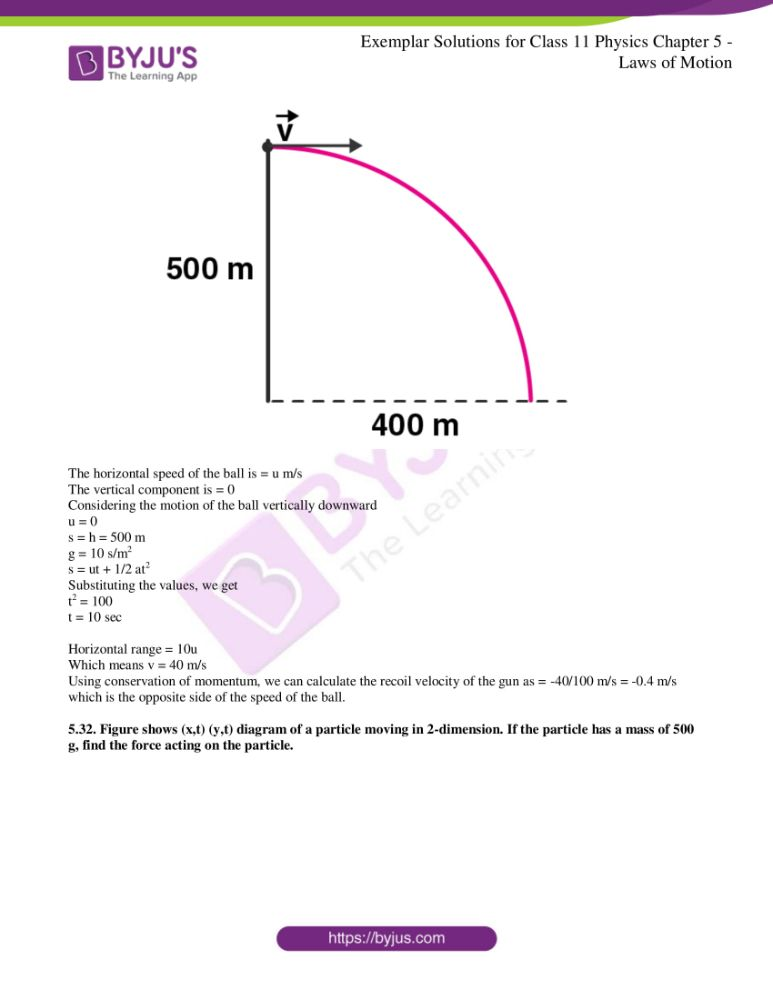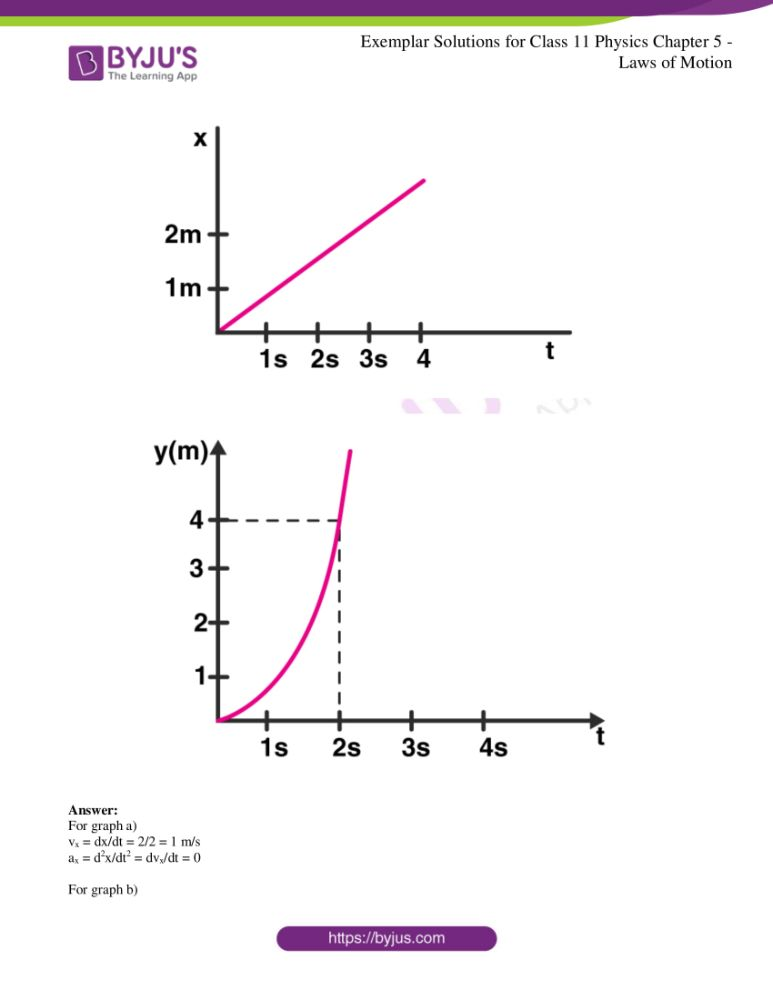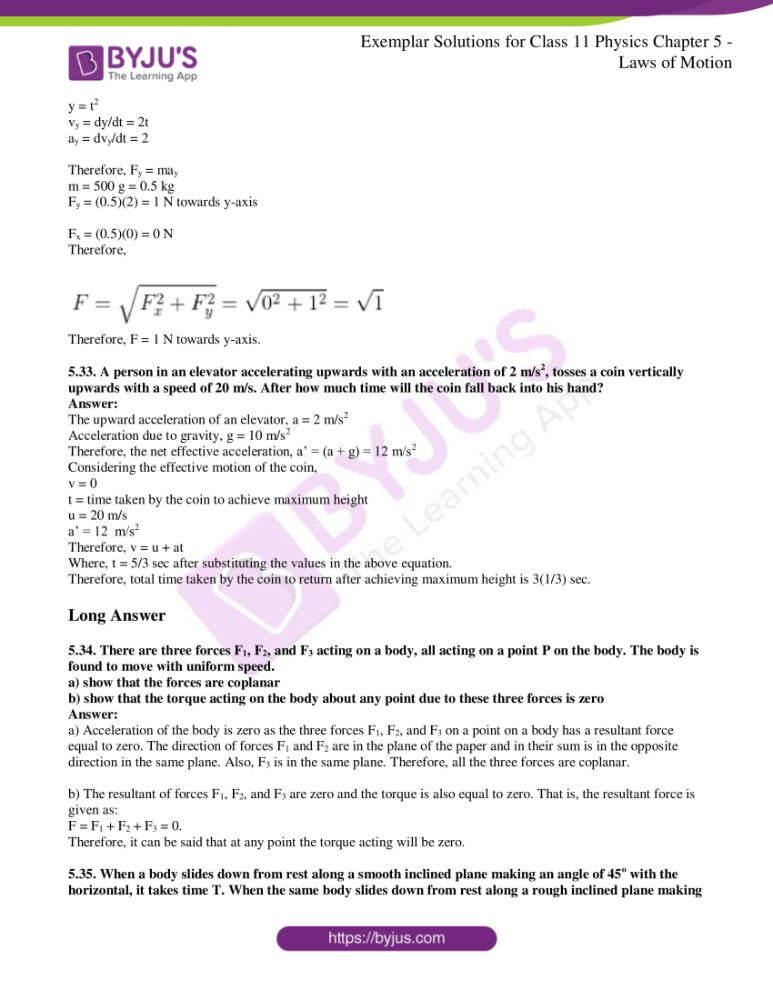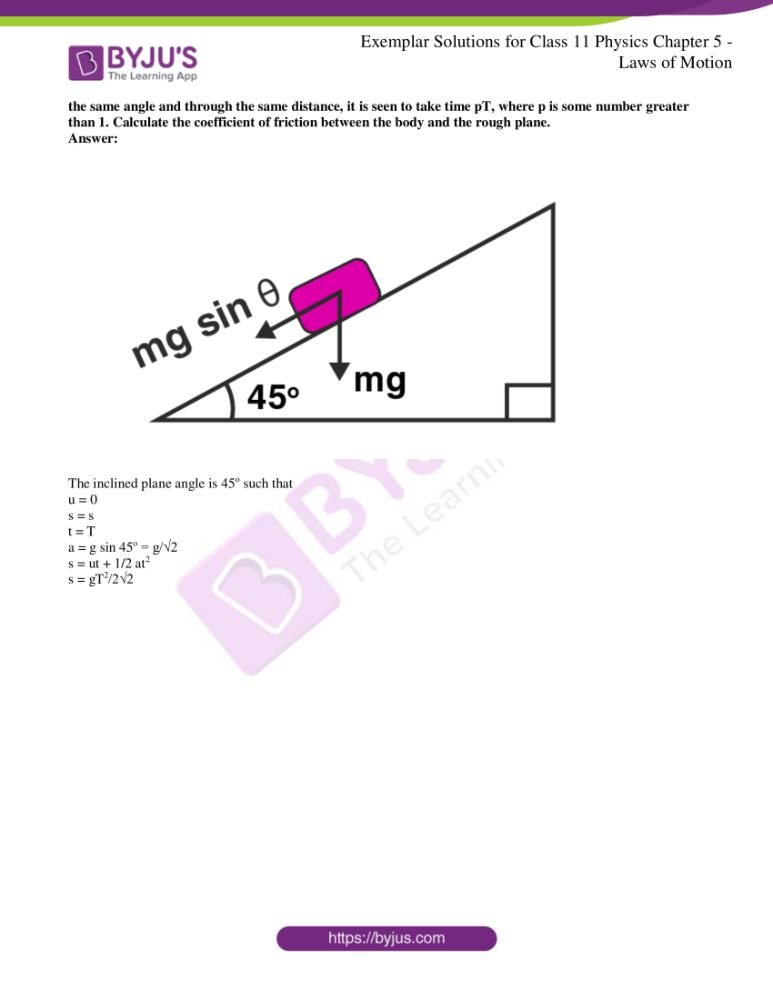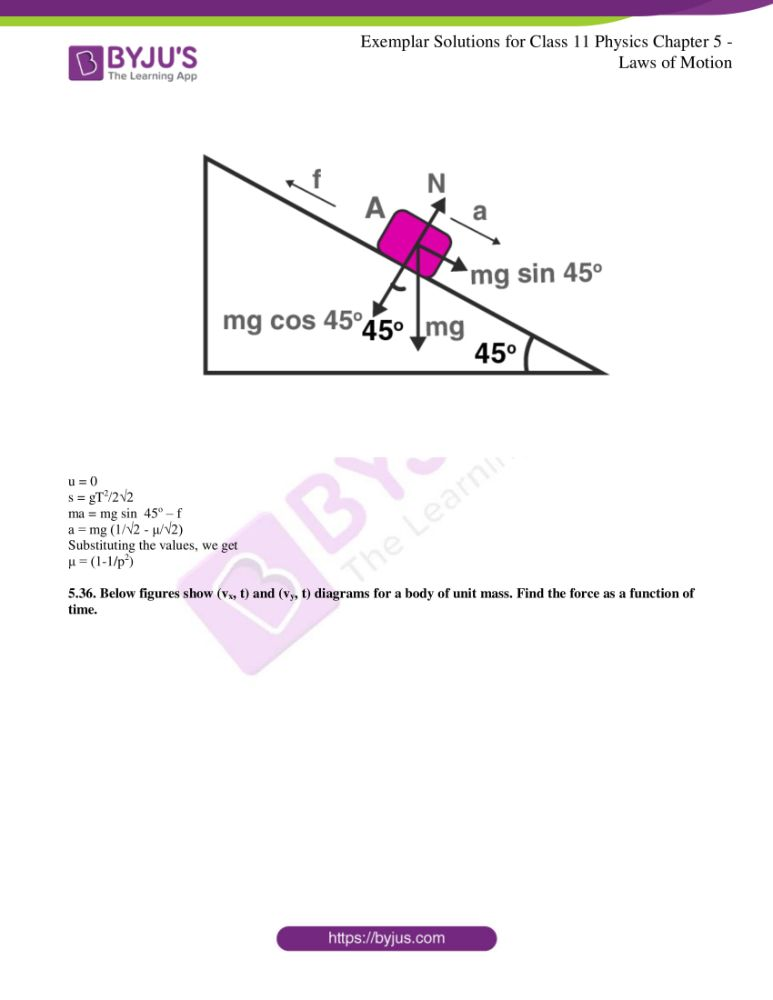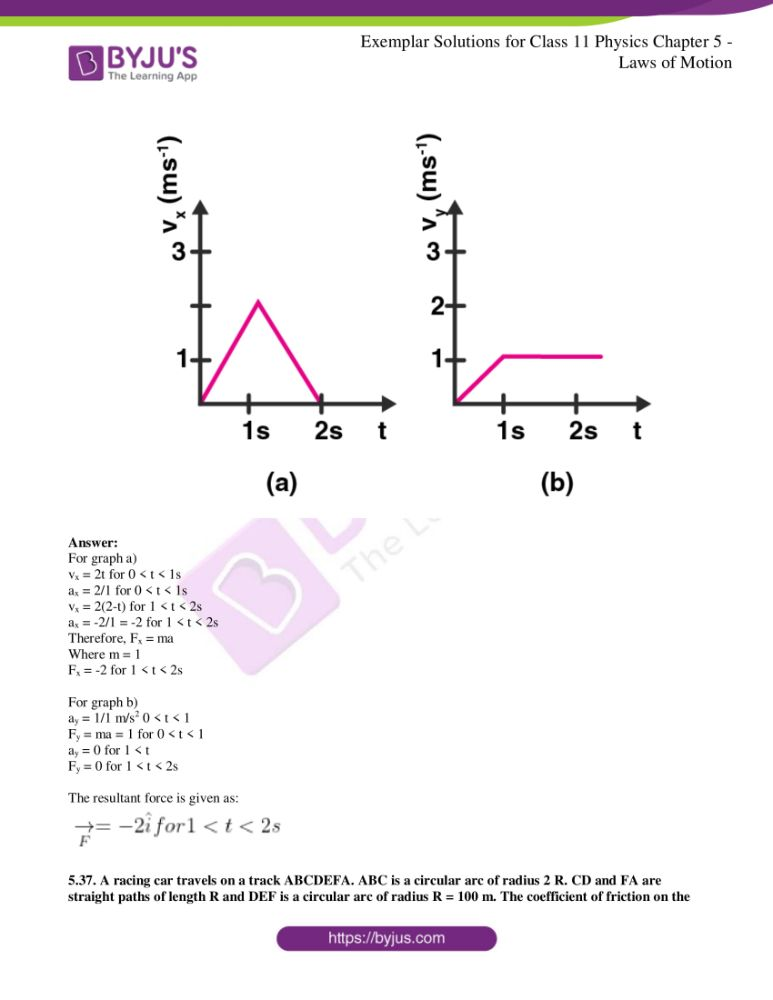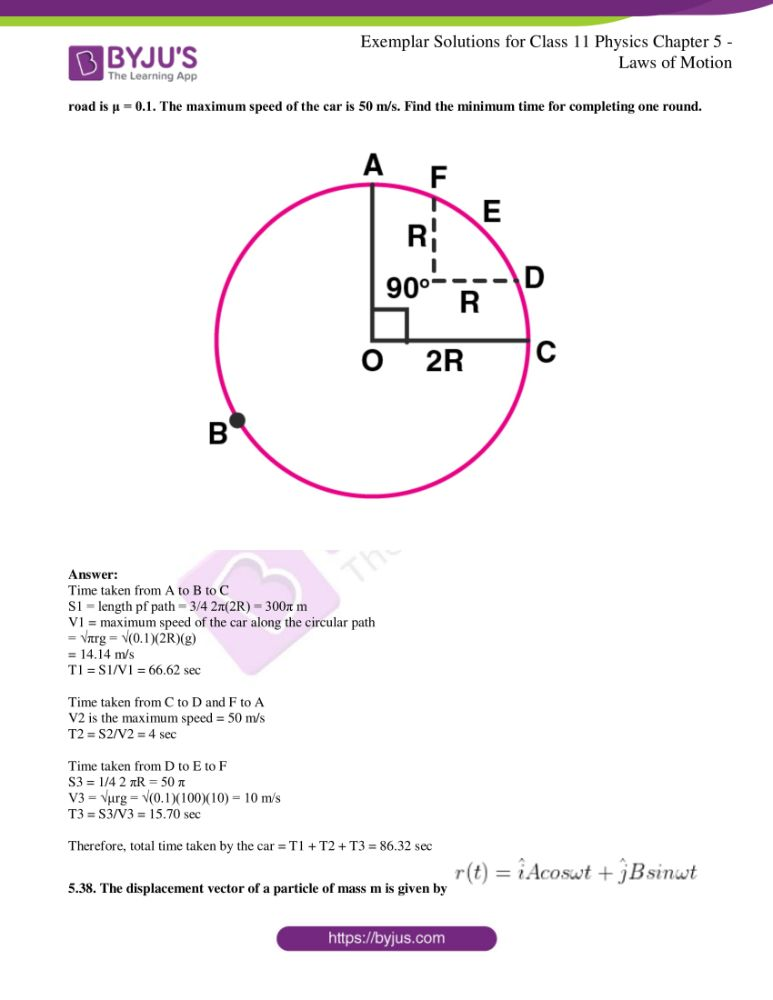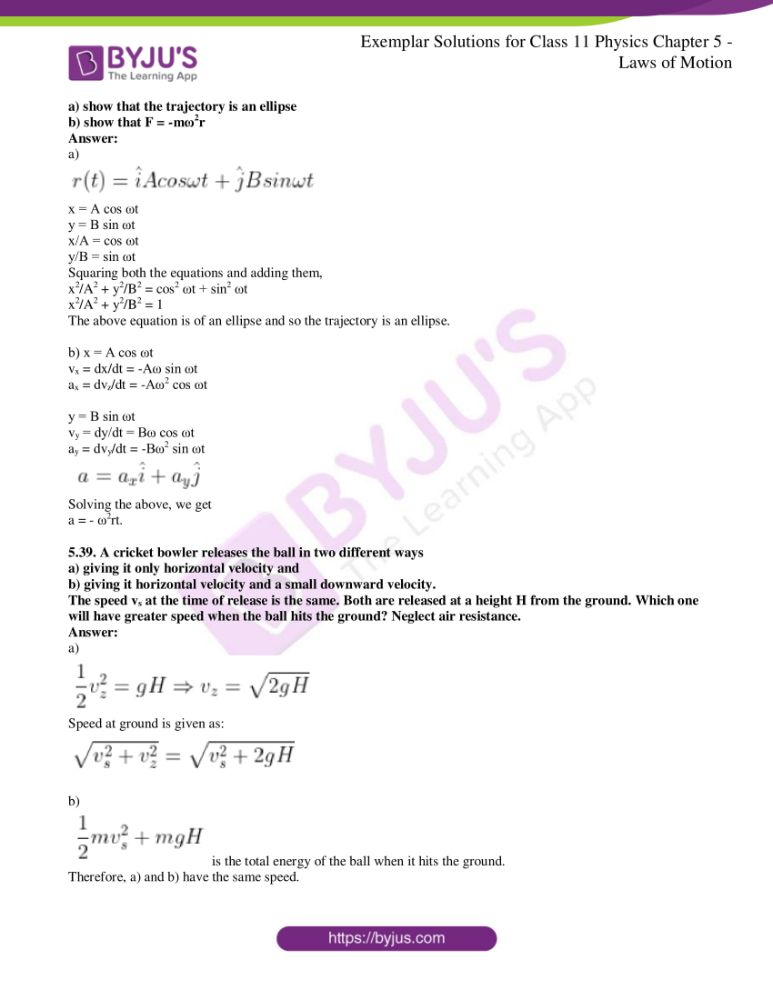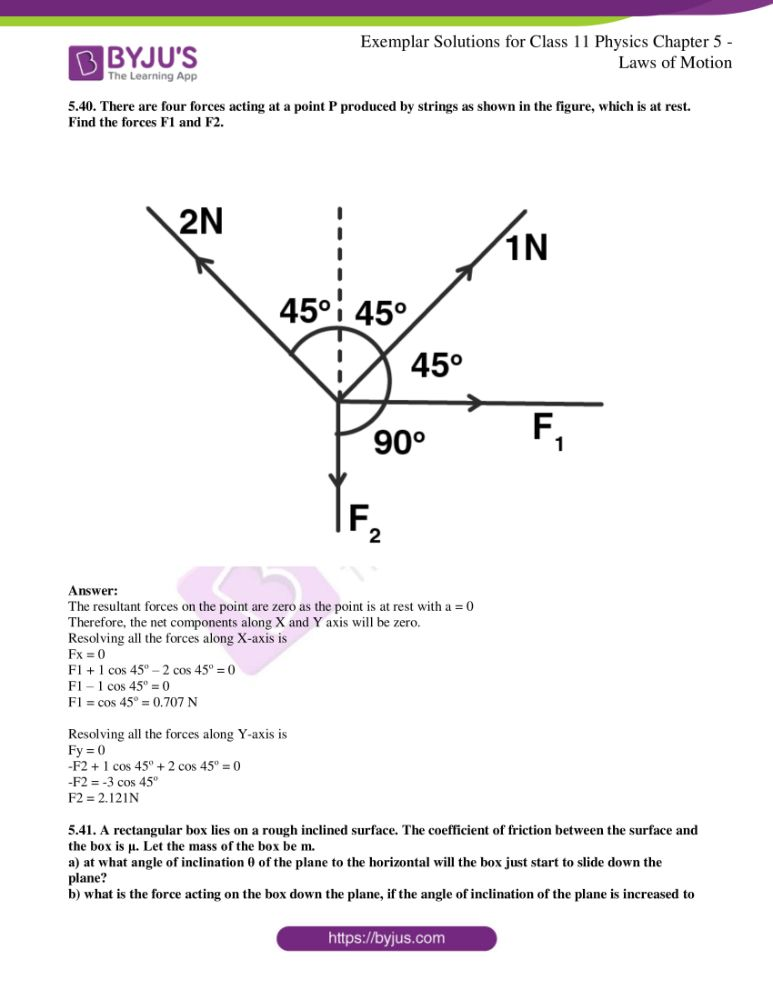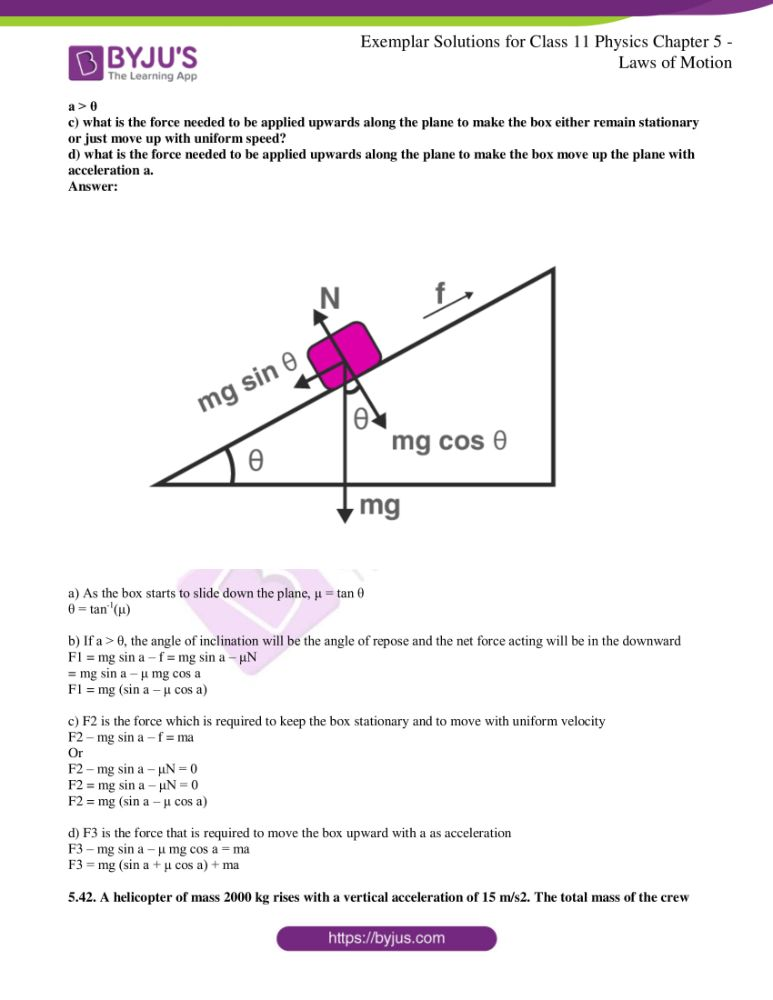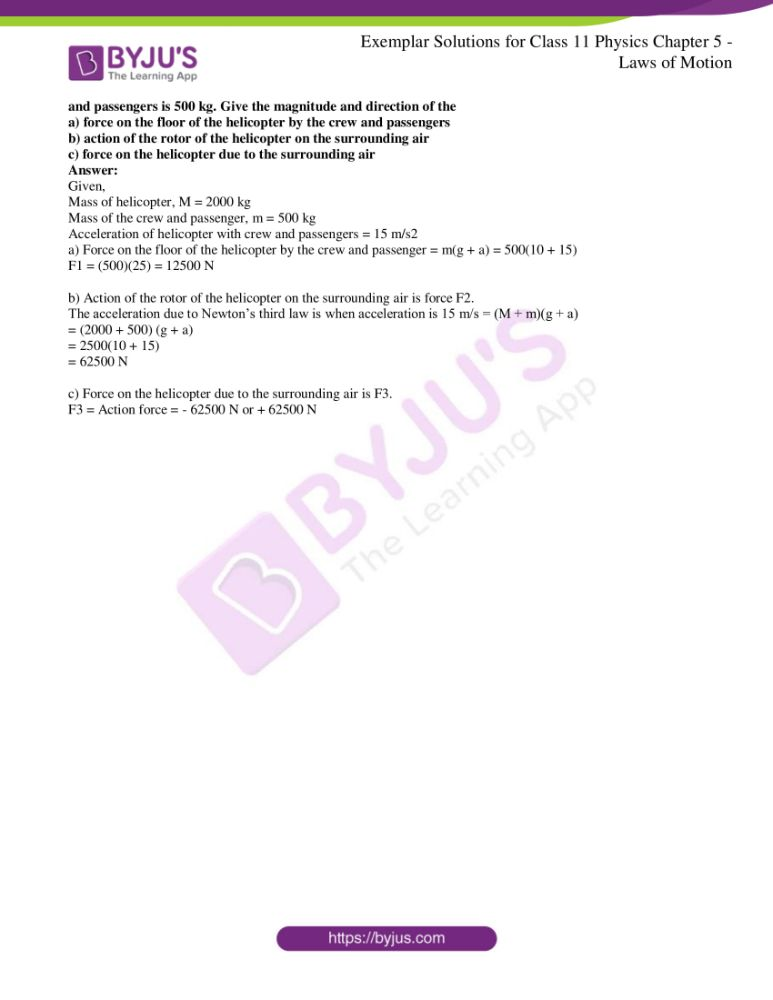### Multiple Choice Questions I

5.1. A ball is travelling with uniform translator motion. This means that

a) it is at rest

b) the path can be a straight line or circular and the ball travels with uniform speed

c) all parts of the ball have the same velocity and the velocity is constant

d) the centre of the ball moves with constant velocity and the ball spins about its centre uniformly

The correct option is c) all parts of the ball have the same velocity and the velocity is constant

5.2. A metre scale is moving with uniform velocity. This implied

a) the force acting on the scale is zero, but a torque about the centre of mass can act on the scale

b) the force acting on the scale is zero and the torque acting about the centre of mass of the scale is also zero

c) the total force acting on it need not be zero but the torque on it is zero

d) neither the force nor the torque needs to be zero

The correct answer is b) the force acting on the scale is zero and the torque acting about the centre of mass of the scale is also zero

5.3. A cricket ball of mass 150 g has an initial velocity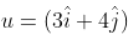m/s and a final velocity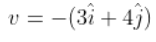m/s after being hit. The change in momentum

a) zero

b)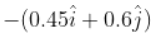c)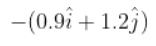d)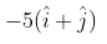The correct answer is c)5.4. In the previous problem, the magnitude of the momentum transferred during the hit is

a) zero

b) 0.75 kg.m/s

c) 1.5 kg.m/s

d) 14 kg.m/s

The correct option is c) 1.5 kg.m/s

5.5. Conservation of momentum in a collision between particles can be understood from

a) conservation of energy

b) Newton’s first law only

c) Newton’s second law only

d) both Newton’s second and third law

The correct answer is d) both Newton’s second and third law

5.6. A hockey player is moving northward and suddenly turns westward with the same speed to avoid an opponent. The force that acts on the player is

a) frictional force along westward

b) muscle force along southward

c) frictional force along south-west

d) muscle force along south-west

The correct answer is c) frictional force along the south-west

5.7. A body of mass 2 kg travels according to the law x(t) = p(t) + qt2 + rt3 where p = 3 m/s, q = 4 m/s2, and r = 5 m/s3. The force acting on the body at t=2 seconds is

a) 136 N

b) 134 N

c) 158 N

d) 68 N

The correct answer is a) 136 N

5.8. A body with mass 5 kg is acted upon by a force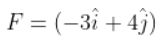N. If its initial velocity at t = 0 is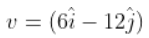m/s, the time at which it will just have a velocity along the y-axis is

a) never

b) 10 s

c) 2 s

d) 15 s

The correct answer is b) 10 s

5.9. A car of mass m starts from rest and acquires a velocity along the east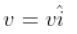in two seconds. Assuming the car moves with uniform acceleration, the force exerted on the car is

a) mv/2 eastward and is exerted by the car engine

b) mv/2 eastward and is due to the friction on the tyres exerted by the road

c) more than mv/2 eastward exerted due to the engine and overcomes the friction of the road

d) mv/2 exerted by the engine

The correct answer is b) mv/2 eastward and is due to the friction on the tyres exerted by the road

### Multiple Choice Questions II

5.10. The motion of a particle of mass m is given by x = 0 for t < 0 sec, x(t) = A sin 4p t for 0 < t < (1/4) sec, and x = 0 for t > (1/4) sec. Which of the following statements is true?

a) the force at t = (1/8) sec on the particle is -16π2Am

b) the particle is acted upon by on impulse of magnitude 4π2Am at t = 0 sec and t = (1/4) sec

c) the particle is not acted upon by any force

d) the particle is not acted upon by a constant force

e) there is no impulse acting on the particle

a) the force at t = (1/8) sec on the particle is -16π2Am

b) the particle is acted upon by on impulse of magnitude 4π2Am at t = 0 sec and t = (1/4) sec

d) the particle is not acted upon by a constant force

5.11. In figure, the coefficient of friction between the floor and the body B is 0.1. The coefficient of friction between the bodies B and A is 0.2. A force F is applied as shown on B. The mass of A is m/2 and of B is m. Which of the following statements are true?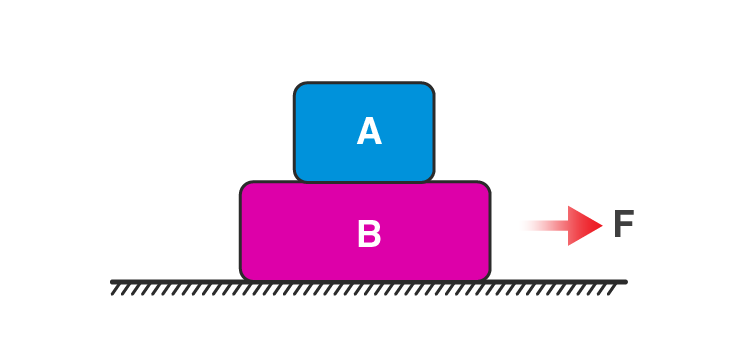a) the bodies will move together if F = 0.25 mg

b) the body A will slip with respect to B if F = 0.5 mg

c) the bodies will move together if F = 0.5 mg

d) the bodies will be at rest if F = 0.1 mg

e) the maximum value of F for which the two bodies will move together is 0.45 mg

a) the bodies will move together if F = 0.25 mg

b) the body A will slip with respect to B if F = 0.5 mg

d) the bodies will be at rest if F = 0.1 mg

e) the maximum value of F for which the two bodies will move together is 0.45 mg

5.12. Mass m1 moves on a slope making an angle θ with the horizontal and is attached to mass m2 by a string passing over a frictionless pulley as shown in the figure. The coefficient of friction between m1 and the sloping surface is μ. Which of the following statements are true?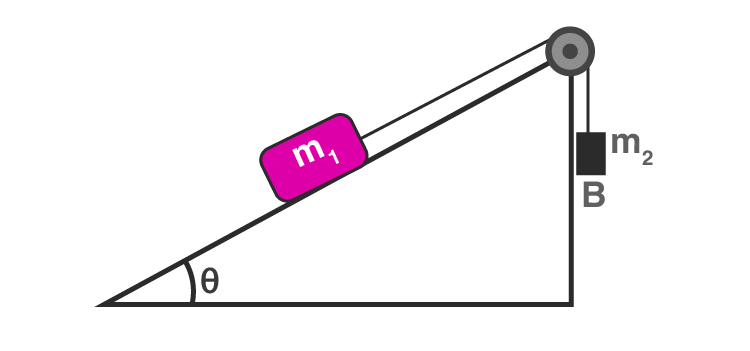a) if m2 > m1 sin θ, the body will move up the plane

b) if m2 > m1(sin θ + μ cos θ), the body will move up the plane

c) if m2 < m1 (sin θ + μ cos θ), the body will move up the plane

d) if m2 < m1 (sin θ – μ cos θ), the body will move down the plane

b) if m2 > m1(sin θ + μ cos θ), the body will move up the plane

d) if m2 < m1 (sin θ – μ cos θ), the body will move down the plane

5.13. In the figure, a body A of mass m slides on a plane inclined at angle θ1 to the horizontal and μ1 is the coefficient of friction between A and the plane. A is connected by a light string passing over a frictionless pulley to another body B, also of mass m, sliding on a frictionless plane inclined at angle θ2 to the horizontal. Which of the following statements are true?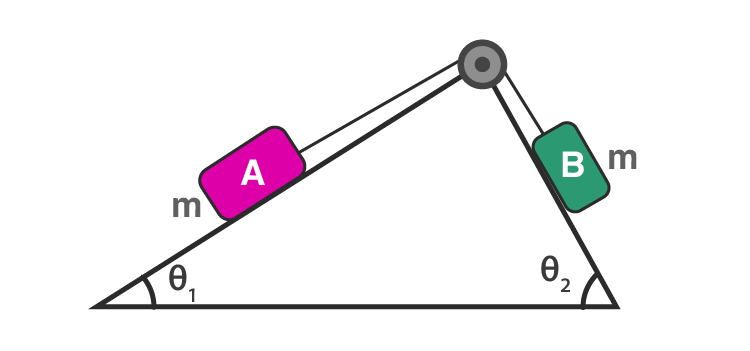a) A will never move up the plane

b) A will just start moving up the plane when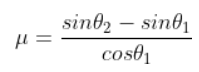c) for A to move up the plane, θ2 must always be greater than θ1

d) B will always slide down with constant speed

b) A will just start moving up the plane whenc) for A to move up the plane, θ2 must always be greater than θ1

5.14. Two billiard balls A and B, each of mass 50 g and moving in opposite directions with speed of 5 m/s each, collide and rebound with the same speed. If the collision lasts for 10-3 seconds, which of the following statements are true?

a) the impulse imparted to each ball is 0.25 kg.m/s and the force on each ball is 250N

b) the impulse imparted to each ball is 0.25 kg.m/s and the force exerted on each ball is 25 × 10-5 N

c) the impulse imparted to each ball is 0.5 Ns

d) the impulse and the force on each ball are equal in magnitude and opposite in direction

c) the impulse imparted to each ball is 0.5 Ns

d) the impulse and the force on each ball are equal in magnitude and opposite in direction

5.15. A body of mass 10 kg is acted upon by two perpendicular forces, 6N and 8N. The resultant acceleration of the body is

a) 1 m/s2 at an angle of tan-1 (4/3) w.r.t 6N force

b) 0.2 m/s2 at an angle of tan-1 (4/3) w.r.t 6N force

c) 1 m/s2 at an angle of tan-1(3/4) w.r.t 8N force

d) 0.2 m/s2 at an angle of tan-1(3/4) w.r.t 8N force

a) 1 m/s2 at an angle of tan-1 (4/3) w.r.t 6N force

c) 1 m/s2 at an angle of tan-1(3/4) w.r.t 8N force

5.16. A girl riding a bicycle along a straight road with a speed of 5 m/s throws a stone of mass 0.5 kg which has a speed of 15 m/s with respect to the ground along her direction of motion. The mass of the girl and the bicycle is 50 kg. Does the speed of the bicycle change after the stone is thrown? What is the change in speed, if so?

Given,

m1 = 50 kg

m2 = 0.5 kg

u1 = 5 m/s

u2 = 5 m/s

v1 = ?

v2 = 15 m/s

From the law of conservation of momentum,

Initial momentum = final momentum

(m1 + m2) u1 = m1v1 + m2v2

Substituting the values, we get

v1 = 4.9 m/s

Therefore, the speed of cycle and the girl decreases by: 5 – 4.9 = 0.1 m/s.

5.17. A person of mass 50 kg stands on a weighing scale on a lift. If the lift is descending with a downward acceleration of 9 m/s2 what would be the reading of the weighing scale?

The apparent weight on the weighing scale decreases when the lift descends with an acceleration a.

Let W’ be the apparent weight

Therefore,

W’ = R = (mg – ma) = m(g – a)

Therefore, apparent weight, W’ = 50(10-9) = 50 N

Reading of the weighing scale = R/g = 50/10 = 5 kg.

5.18. The position time graph of a body of mass 2 kg is as given in the figure. What is the impulse on the body at t – 0 sec and t = 4 sec.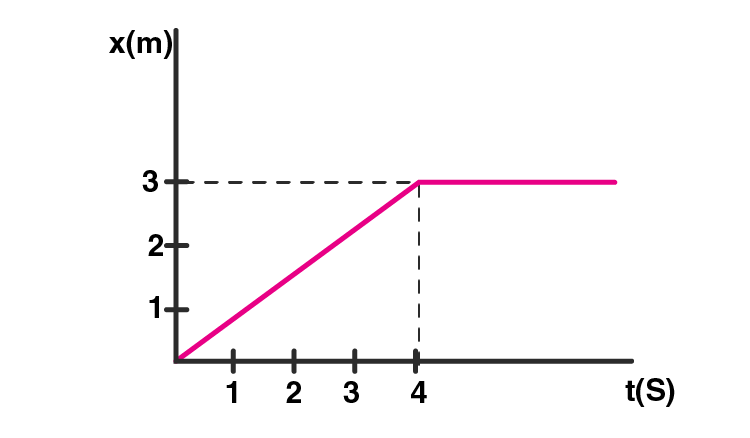Mass of body, m = 2 kg

Time, t = 0

Initial velocity, v1 = 0

From graph, we know that

t ≥ 0 to t ≤ 4 which is a straight line.

The velocity of the body is constant

v2 = tan θ = 3/4 = 0.75 m/s

At t ≥ 4, the slope of the graph is zero so velocity v3 = 0

Impulse = Ft = (dp/dt)dt = dp

Impulse = change in momentum

Impulse at t = 0 is given as:

2(0.75 – 0) = 1.50 kg.m/s

Impulse at t = 4 is given as:

m(v3 – v2) = 2(0-0.75) = -1.50 kg.m/s

Therefore, impulse at t = 0 increases by +1.5 kg.m/s and at t = 4 it decreases by -1.5 kg.m/s.

5.19. A person driving a car suddenly applies the brakes on seeing a child on the road ahead. If he is not wearing a seat belt, he falls forward and hits his head against the steering wheel. Why?

When a person applies brakes suddenly, he tends to fall forward and hit his head against the steering wheel if he is not wearing the seat belt because his upper body continues to be in a state of motion and in the same direction as that of the car which is due to inertia of motion.

5.20. The velocity of a body of mass 2 kg as a function of t is given by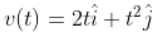Find the momentum and the force acting on it at time t = 2 sec.

m = 2 kg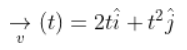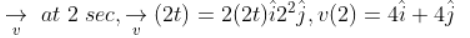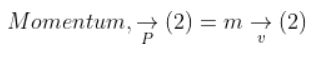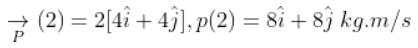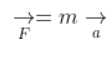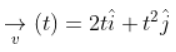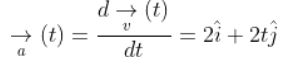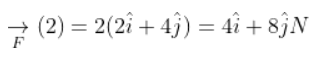5.21. A block placed on a rough horizontal surface is pulled by a horizontal force F. Let f be the force applied by the rough surface on the block. Plot a graph of f versus F.

F1 is the force that is acting on the heavy box which is opposed by the smaller frictional force f1 and is equal to F1. For box to move, F = Fs which is the maximum static frictional force. This force is also corresponding to the frictional force fs on the y-axis.

The frictional force decreases when Fk < Fs is applied on the body and it starts to move such the less friction is fk < fs.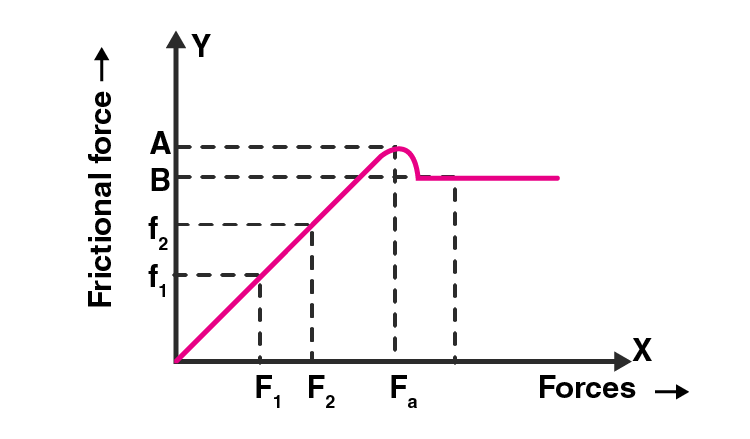5.22. Why are porcelain objects wrapped in paper or straw before packing for transportation?

Porcelain objects are wrapped in paper or straw before packing for transportation because they fragile in nature. While transporting these objects change in velocity might bring cracks on them when the acceleration (v – u)/t decreases. To avoid the force on these objects, they are wrapped with paper.

5.23. Why does a child feel more pain when falls down on a hard cement floor, than when she falls on the soft muddy ground in the garden?

When a child falls on a hard cement floor, the child feels more pain than when the child falls on the soft muddy ground in the garden because the time taken by the child to stop is very small on the cemented ground whereas, on the soft muddy ground, the time taken is more. When the time taken to stop is more, the acceleration is decreased resulting in reduced force.

5.24. A woman throws an object of mass 500 g with a speed of 25 m/s.

a) what is the impulse imparted to the object?

b) if the object hits a wall and rebounds with half the original speed, what is the change in momentum of the object?

a) Mass of the object, m = 500 g = 0.5 kg

u = 0

v = 25 m/s

Impulse is given as: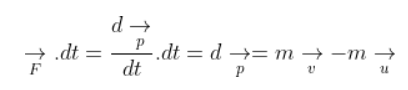Substituting the values, we get impulse = 12.5 N.s

b) m = 0.5 kg

u = +25 m/s

v = -25/2 m/s

∆p = m(v – u) = -18.75 N.s

5.25. Why are mountain roads generally made winding upwards rather than going straight up?

The mountain roads are generally made winding upwards rather than going straight up because the force of friction is high which reduces the chances of skidding. When the roads are straight up, the value of frictional force increases which increases the chance of skidding. This is given by:

fs = μN cosθ where θ is the angle of inclination.

5.26. A mass of 2 kg is suspended with thread AB. Thread CD of the same type is attached to the other end of 2 kg mass. Lower thread is pulled gradually harder and harder in the downward direction so as to apply force on AB. Which of the threads will break and why?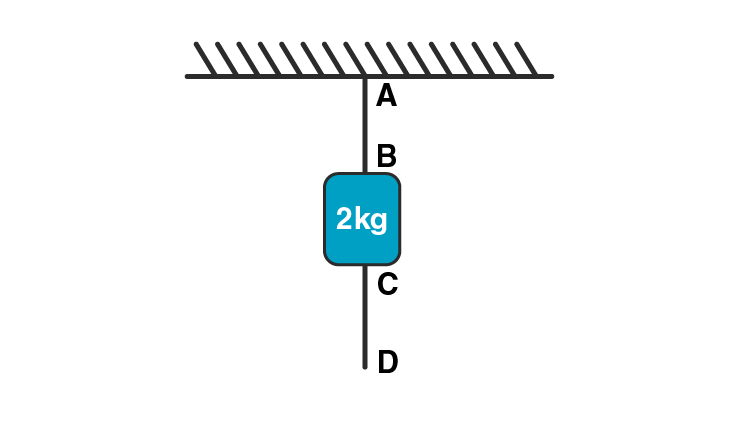The force acting on the thread AB is equal to the force F as the mass 2 kg is acting downward. So, it can be said that the force acting on the AB is 2 kg more than on D and therefore, the thread AB breaks.

5.27. In the above-given problem if the lower thread is pulled with a jerk, what happens?

When the lower thread is pulled with a jerk, the thread CD breaks the thread is under the inertia of rest and the mass 2 kg above acts on it.

5.28. Two masses of 5 kg and 3 kg are suspended with help of massless inextensible strings as shown in the figure. Calculate T1 and T2 when whole system is going upwards with acceleration = 2 m/s2.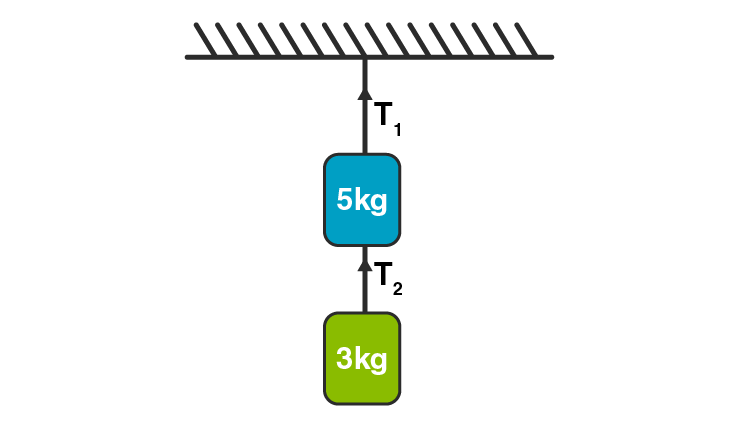The acceleration of the whole system is, a = 2 m/s2

m1 = 5 kg

m2 = 3 kg

g = 9.8 m/s2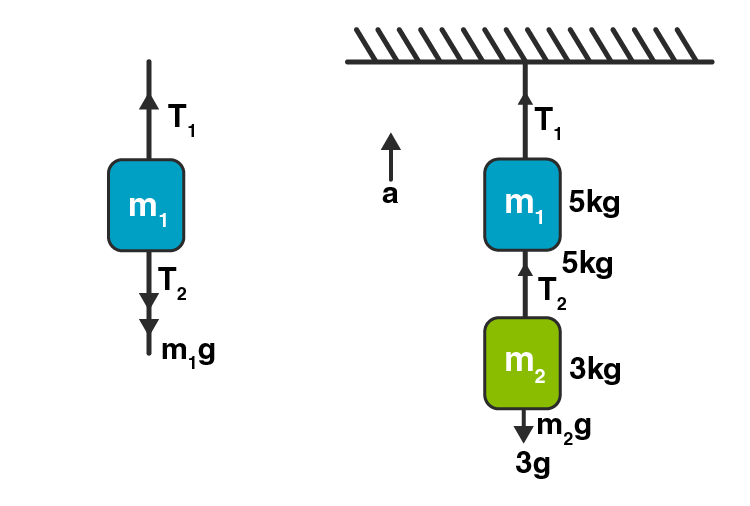Tension in a string is equal and opposite in all parts of the string.

Forces on mass m1 is

T1 – T2 – m1g = m2a

T1 – T2 – 5g = 5a

T1 – T2 = 5g + 5a

T1 – T2 = 5(9.8 + 2)

T1 – T2 = 59 N

Force on mass m2 is

T2 – m2g = m2a

T2 = m2(g + a) = 3 (9.8 + 2) = 35.4

T1 = 94.4 N

5.29. Block A of weight 100N rests on a frictionless inclined plane of slope angle 30o. A flexible cord attached to A passes over a frictionless pulley and is connected to block B of weight W. Find the weight W for which the system is in equilibrium.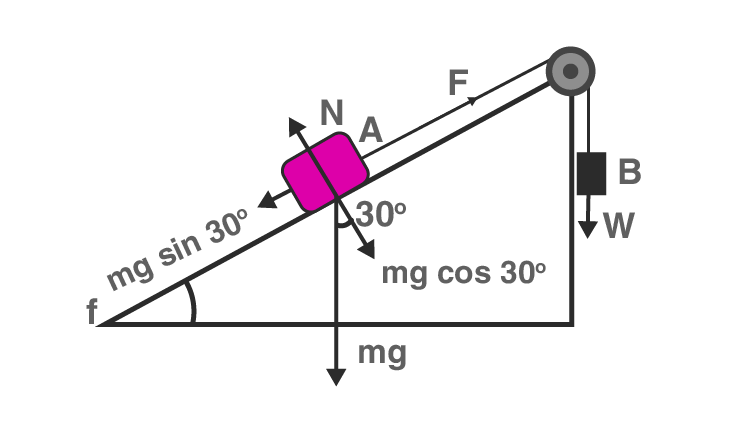When there is equilibrium between A or B,

mg sin 30o = F

1/2 mg = F

F = (1/2)(100) = 50

Therefore, when B is at rest, W = F = 50 N.

5.30. A bock of mass M is held against a rough vertical wall by pressing it with a finger. If the coefficient of friction between the block and the wall is μ and the acceleration due to gravity is g, calculate the minimum force required to be applied by the ginger to hold the block against the wall?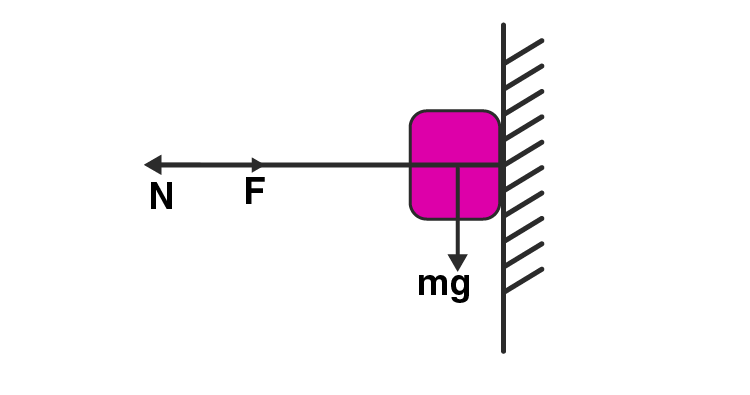F is the force applied by the finger on the body whose mass is M and the body is at rest against the wall.

Using the balanced condition,

F = N

f = Mg

μF = Mg

F = Mg/μ is the minimum force that is required to hold the block against the wall at rest.

5.31. A 100 kg gun fires a ball of 1 kg horizontally from a cliff of height 500 m. It falls on the ground at a distance of 400 m from the bottom of the cliff. Find the recoil velocity of the gun.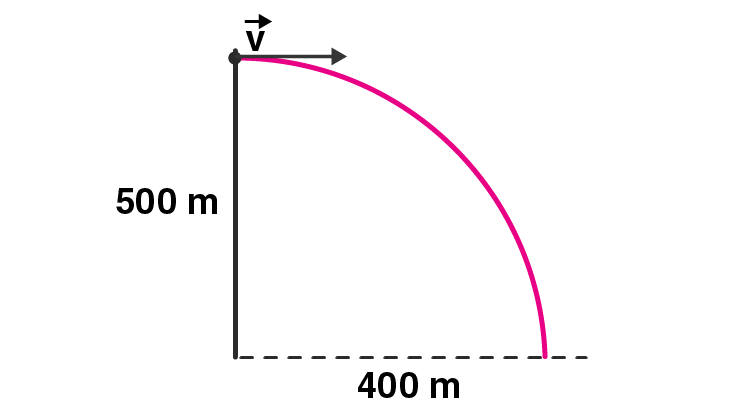The horizontal speed of the ball is = u m/s

The vertical component is = 0

Considering the motion of the ball vertically downward

u = 0

s = h = 500 m

g = 10 s/m2

s = ut + 1/2 at2

Substituting the values, we get

t2 = 100

t = 10 sec

Horizontal range = 10u

Which means v = 40 m/s

Using conservation of momentum, we can calculate the recoil velocity of the gun as = -40/100 m/s = -0.4 m/s which is the opposite side of the speed of the ball.

5.32. Figure shows (x,t) (y,t) diagram of a particle moving in 2-dimension. If the particle has a mass of 500 g, find the force acting on the particle.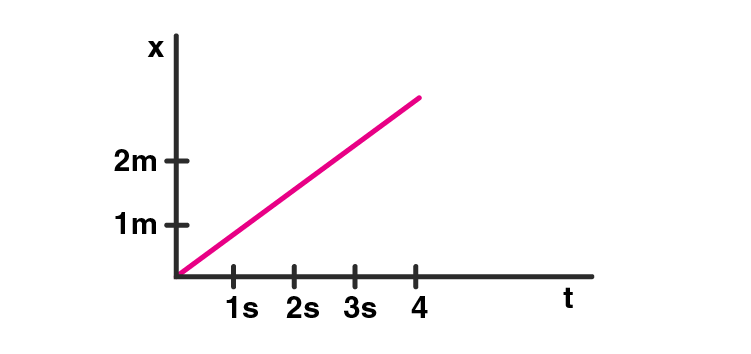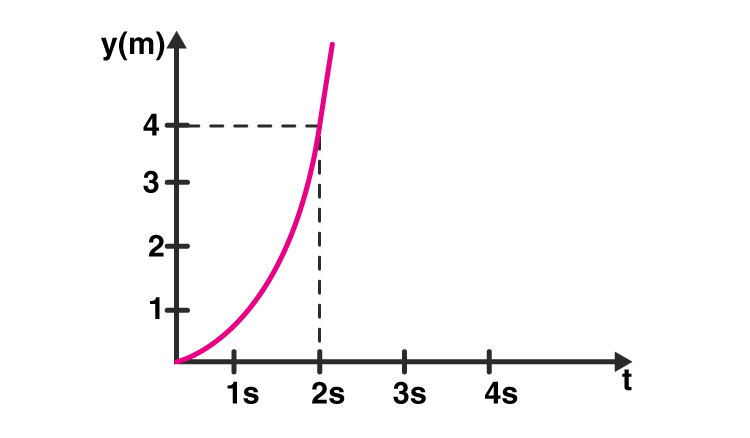For graph a)

vx = dx/dt = 2/2 = 1 m/s

ax = d2x/dt2 = dvx/dt = 0

For graph b)

y = t2

vy = dy/dt = 2t

ay = dvy/dt = 2

Therefore, Fy = may

m = 500 g = 0.5 kg

Fy = (0.5)(2) = 1 N towards y-axis

Fx = (0.5)(0) = 0 N

Therefore,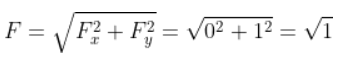Therefore, F = 1 N towards y-axis.

5.33. A person in an elevator accelerating upwards with an acceleration of 2 m/s2, tosses a coin vertically upwards with a speed of 20 m/s. After how much time will the coin fall back into his hand?

The upward acceleration of an elevator, a = 2 m/s2

Acceleration due to gravity, g = 10 m/s2

Therefore, the net effective acceleration, a’ = (a + g) = 12 m/s2

Considering the effective motion of the coin,

v = 0

t = time taken by the coin to achieve maximum height

u = 20 m/s

a’ = 12 m/s2

Therefore, v = u + at

Where, t = 5/3 sec after substituting the values in the above equation.

Therefore, the total time taken by the coin to return after achieving maximum height is 3(1/3) sec.

5.34. There are three forces F1, F2, and F3 acting on a body, all acting on a point P on the body. The body is found to move with uniform speed.

a) show that the forces are coplanar

b) show that the torque acting on the body about any point due to these three forces is zero

a) Acceleration of the body is zero as the three forces F1, F2, and F3 on a point on a body has a resultant force equal to zero. The direction of forces F1 and F2 are in the plane of the paper and in their sum is in the opposite direction in the same plane. Also, F3 is in the same plane. Therefore, all the three forces are coplanar.

b) The resultant of forces F1, F2, and F3 are zero and the torque is also equal to zero. That is, the resultant force is given as:

F = F1 + F2 + F3 = 0.

Therefore, it can be said that at any point the torque acting will be zero.

5.35. When a body slides down from rest along a smooth inclined plane making an angle of 45o with the horizontal, it takes time T. When the same body slides down from rest along a rough inclined plane making the same angle and through the same distance, it is seen to take time pT, where p is some number greater than 1. Calculate the coefficient of friction between the body and the rough plane.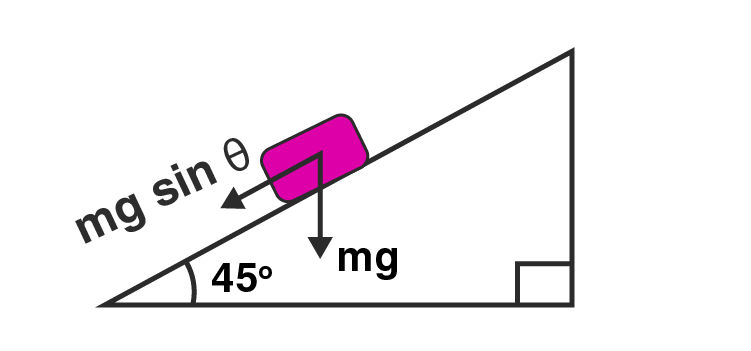The inclined plane angle is 45o such that

u = 0

s = s

t = T

a = g sin 45o = g/√2

s = ut + 1/2 at2

s = gT2/2√2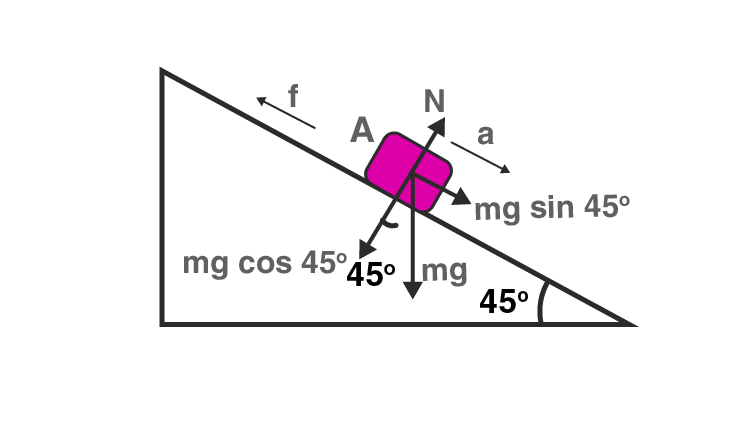u = 0

s = gT2/2√2

ma = mg sin 45o – f

a = mg (1/√2 – μ/√2)

Substituting the values, we get

μ = (1-1/p2)

5.36. Below figures show (vx, t) and (vy, t) diagrams for a body of unit mass. Find the force as a function of time.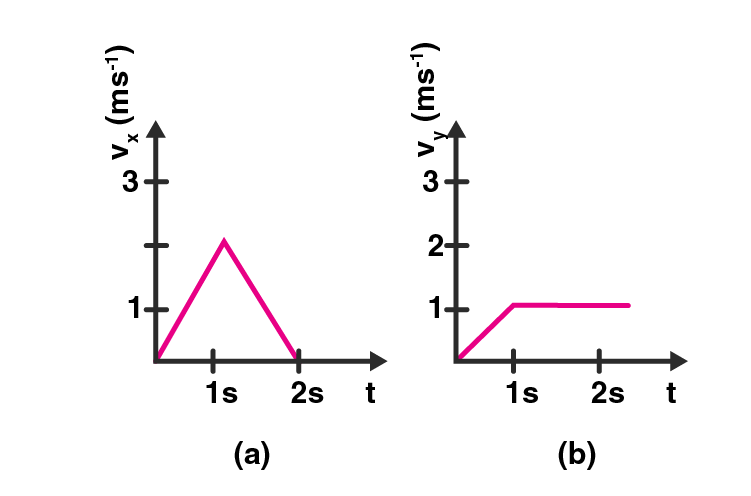For graph a)

vx = 2t for 0 < t < 1s

ax = 2/1 for 0 < t < 1s

vx = 2(2-t) for 1 < t < 2s

ax = -2/1 = -2 for 1 < t < 2s

Therefore, Fx = ma

Where m = 1

Fx = -2 for 1 < t < 2s

For graph b)

ay = 1/1 m/s2 0 < t < 1

Fy = ma = 1 for 0 < t < 1

ay = 0 for 1 < t

Fy = 0 for 1 < t < 2s

The resultant force is given as: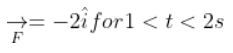5.37. A racing car travels on a track ABCDEFA. ABC is a circular arc of radius 2 R. CD and FA are straight paths of length R and DEF is a circular arc of radius R = 100 m. The coefficient of friction on the road is μ = 0.1. The maximum speed of the car is 50 m/s. Find the minimum time for completing one round.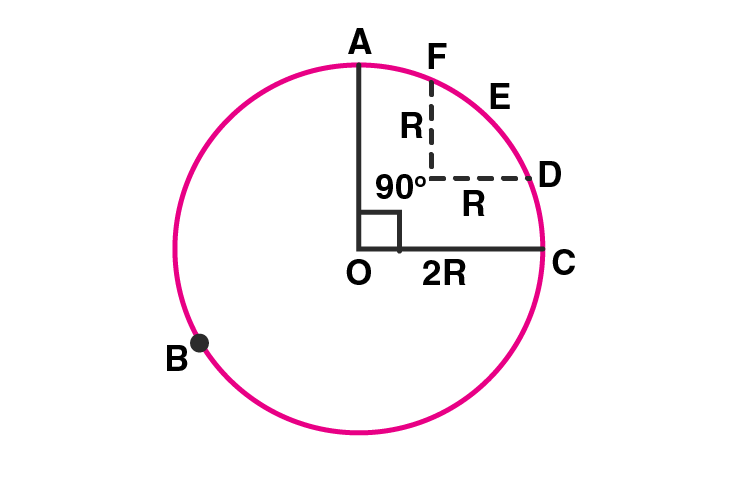Time taken from A to B to C

S1 = length pf path = 3/4 2π(2R) = 300π m

V1 = maximum speed of the car along the circular path

= √πrg = √(0.1)(2R)(g)

= 14.14 m/s

T1 = S1/V1 = 66.62 sec

Time taken from C to D and F to A

V2 is the maximum speed = 50 m/s

T2 = S2/V2 = 4 sec

Time taken from D to E to F

S3 = 1/4 2 πR = 50 π

V3 = √μrg = √(0.1)(100)(10) = 10 m/s

T3 = S3/V3 = 15.70 sec

Therefore, total time taken by the car = T1 + T2 + T3 = 86.32 sec

5.38. The displacement vector of a particle of mass m is given by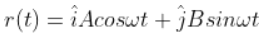a) show that the trajectory is an ellipse

b) show that F = -mω2r

a)x = A cos ωt

y = B sin ωt

x/A = cos ωt

y/B = sin ωt

Squaring both the equations and adding them,

x2/A2 + y2/B2 = cos2 ωt + sin2 ωt

x2/A2 + y2/B2 = 1

The above equation is of an ellipse and so the trajectory is an ellipse.

b) x = A cos ωt

vx = dx/dt = -Aω sin ωt

ax = dvz/dt = -Aω2 cos ωt

y = B sin ωt

vy = dy/dt = Bω cos ωt

ay = dvy/dt = -Bω2 sin ωt

$$\begin{array}{l}a=a_{x}\widehat{i}+a_{y}\widehat{j}\end{array}$$

Solving the above, we get

a = – ω2rt.

5.39. A cricket bowler releases the ball in two different ways

a) giving it only horizontal velocity and

b) giving it horizontal velocity and a small downward velocity.

The speed vs at the time of release is the same. Both are released at a height H from the ground. Which one will have greater speed when the ball hits the ground? Neglect air resistance.

a)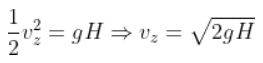Speed at ground is given as: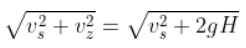b)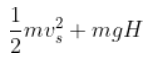is the total energy of the ball when it hits the ground.

Therefore, a) and b) have the same speed.

5.40. There are four forces acting at a point P produced by strings as shown in the figure, which is at rest. Find the forces F1 and F2.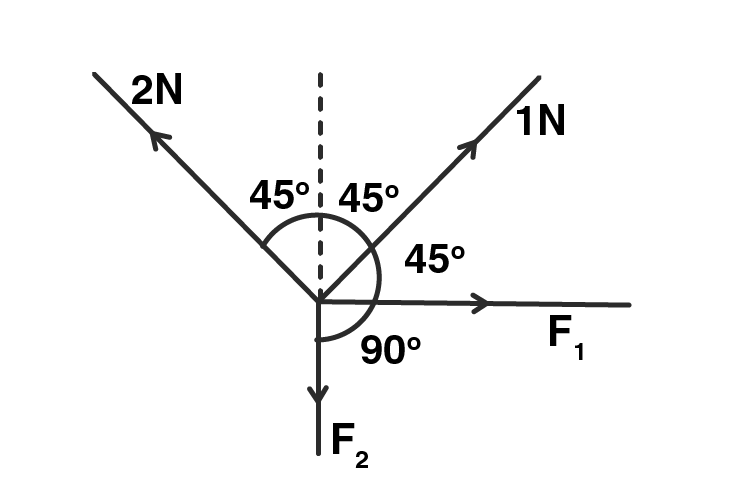The resultant forces on the point are zero as the point is at rest with a = 0

Therefore, the net components along the X and Y axis will be zero.

Resolving all the forces along X-axis is

Fx = 0

F1 + 1 cos 45o – 2 cos 45o = 0

F1 – 1 cos 45o = 0

F1 = cos 45o = 0.707 N

Resolving all the forces along Y-axis is

Fy = 0

-F2 + 1 cos 45o + 2 cos 45o = 0

-F2 = -3 cos 45o

F2 = 2.121N

5.41. A rectangular box lies on a rough inclined surface. The coefficient of friction between the surface and the box is μ. Let the mass of the box be m.

a) at what angle of inclination θ of the plane to the horizontal will the box just start to slide down the plane?

b) what is the force acting on the box down the plane, if the angle of inclination of the plane is increased to a > θ

c) what is the force needed to be applied upwards along the plane to make the box either remain stationary or just move up with uniform speed?

d) what is the force needed to be applied upwards along the plane to make the box move up the plane with acceleration a.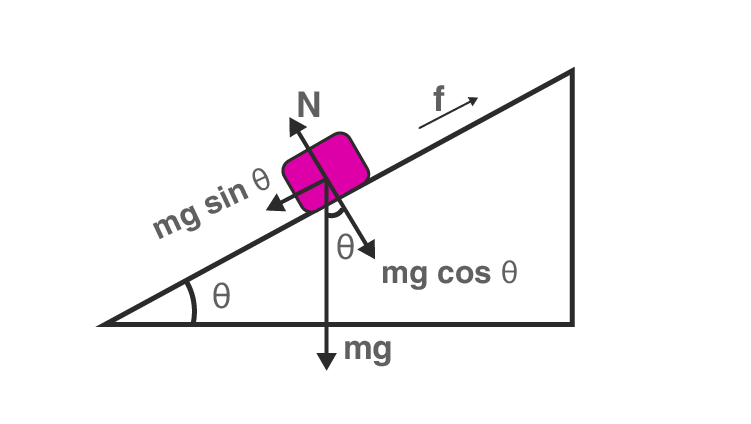a) As the box starts to slide down the plane, μ = tan θ

θ = tan-1(μ)

b) If a > θ, the angle of inclination will be the angle of repose and the net force acting will be in the downward

F1 = mg sin a – f = mg sin a – μN

= mg sin a – μ mg cos a

F1 = mg (sin a – μ cos a)

c) F2 is the force which is required to keep the box stationary and to move with uniform velocity

F2 – mg sin a – f = ma

Or

F2 – mg sin a – μN = 0

F2 = mg sin a – μN = 0

F2 = mg (sin a – μ cos a)

d) F3 is the force that is required to move the box upward with a as acceleration

F3 – mg sin a – μ mg cos a = ma

F3 = mg (sin a + μ cos a) + ma

5.42. A helicopter of mass 2000 kg rises with a vertical acceleration of 15 m/s2. The total mass of the crew and passengers is 500 kg. Give the magnitude and direction of the

a) force on the floor of the helicopter by the crew and passengers

b) action of the rotor of the helicopter on the surrounding air

c) force on the helicopter due to the surrounding air

Given,

Mass of helicopter, M = 2000 kg

Mass of the crew and passenger, m = 500 kg

Acceleration of helicopter with crew and passengers = 15 m/s2

a) Force on the floor of the helicopter by the crew and passenger = m(g + a) = 500(10 + 15)

F1 = (500)(25) = 12500 N

b) Action of the rotor of the helicopter on the surrounding air is force F2.

The acceleration due to Newton’s third law is when acceleration is 15 m/s = (M + m)(g + a)

= (2000 + 500) (g + a)

= 2500(10 + 15)

= 62500 N

c) Force on the helicopter due to the surrounding air is F3.

F3 = Action force = – 62500 N or + 62500 N

### Why Opt for BYJU’S?

BYJU’S provides study materials to all subjects of Class 11 and Class 12 science students. BYJU’S also monitors the progress of the students by giving feedback and counseling periodically. Our experts clear all your doubts while going through NCERT exemplar. Students can also contact our responsive support team to clear their doubts in all the subjects.